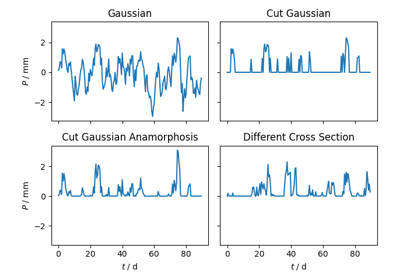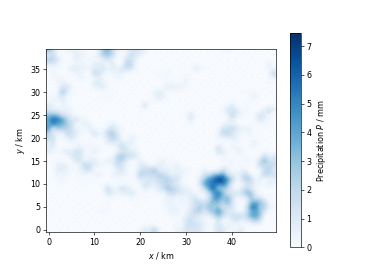# Spatio-Temporal Modeling¶

Spatio-Temporal modelling can provide insights into time dependent processes like rainfall, air temperature or crop yield.

GSTools provides the metric spatio-temporal model for all covariance models by enhancing the spatial model dimension with a time dimension to result in the spatio-temporal dimension `st_dim` and setting a spatio-temporal anisotropy ratio with `st_anis`:

```import gstools as gs
dim = 3  # spatial dimension
st_dim = dim + 1
st_anis = 0.4
st_model = gs.Exponential(dim=st_dim, anis=st_anis)
```

Since it is given in the name “spatio-temporal”, we will always treat the time as last dimension. This enables us to have spatial anisotropy and rotation defined as in non-temporal models, without altering the behavior in the time dimension:

```anis = [0.4, 0.2]  # spatial anisotropy in 3D
angles = [0.5, 0.4, 0.3]  # spatial rotation in 3D
st_model = gs.Exponential(dim=st_dim, anis=anis+[st_anis], angles=angles)
```

In order to generate spatio-temporal position tuples, GSTools provides a convenient function `generate_st_grid`. The output can be used for spatio-temporal random field generation (or kriging resp. conditioned fields):

```pos = dim * [1, 2, 3]  # 3 points in space (1,1,1), (2,2,2) and (3,3,3)
time = range(10)  # 10 time steps
st_grid = gs.generate_st_grid(pos, time)
st_rf = gs.SRF(st_model)
st_field = st_rf(st_grid).reshape(-1, len(time))
```

Then we can access the different time-steps by the last array index.

## Examples¶Gallery generated by Sphinx-Gallery